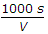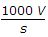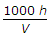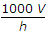# Civil Engineering - UPSC Civil Service Exam Questions

16.

If the diameter of a shaft subjected to torque alone, is doubled, then the horse power P can be increased to

 A. 16 P B. 8 P C. 4 P D. 2 P

Explanation:

No answer description available for this question. Let us discuss.

17.

Which one of the following represents the basic capacity of a single lane road?(where V = speed in kmph, s = Average centre to centre spacing of vehicle in m, h = Average time headway between two vehicles in seconds.)

 A.B.C.D.Explanation:

No answer description available for this question. Let us discuss.

18.

Consider the following factors :
1. Mass of ships to be berthed
2. Overall transit time
3. Speed of berthing
4. Environmental conditions of the port
Which of these factors are taken into account while selecting the type of fender system ?

 A. 1, 2 and 3 B. 2, 3 and 4 C. 1, 3 and 4 D. 1 and 4

Explanation:

No answer description available for this question. Let us discuss.

19.

Which one of the following statements is correct with respect to rigid pavements ?

 A. Vertical stresses are transmitted to the lower layer through the points of contact in granular structure. B. Vertical compressive stress is maximum on the pavement surface directly under the wheel load. C. Lower layers have to take lesser magnitudes of stress D. Stresses on rigid pavements are transmitted to a wider area of sub grade.

Explanation:

No answer description available for this question. Let us discuss.

20.

Consider the following statements :
Selection of the type and grade of the bituminous binder is made on the basis of
1. cost of the binder material.
2. climatic conditions of the area and the construction technique.
3. degree of importance of the road.
4. thickness of pavement layer to be constructed.
Which of these statements is/are correct ?

 A. 1 only B. 2 only C. 1, 2 and 4 D. 1, 2 and 3